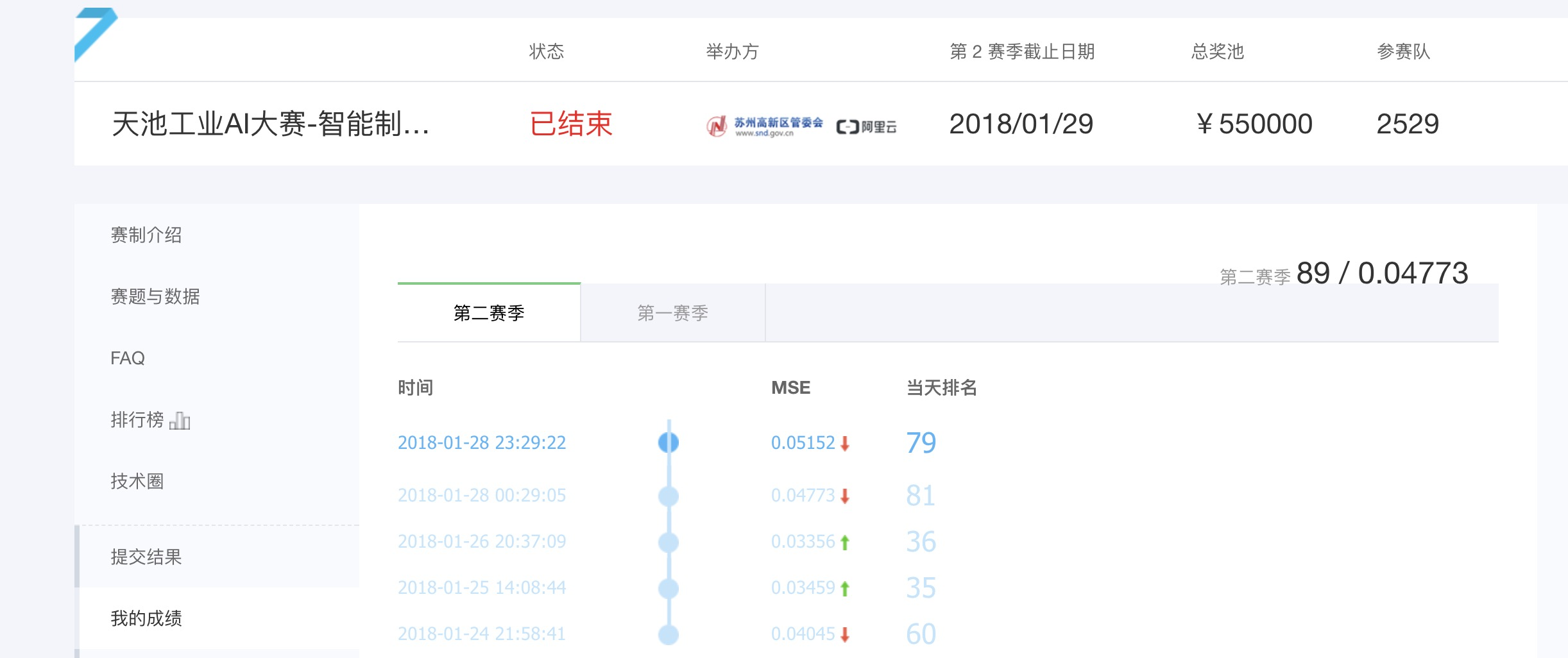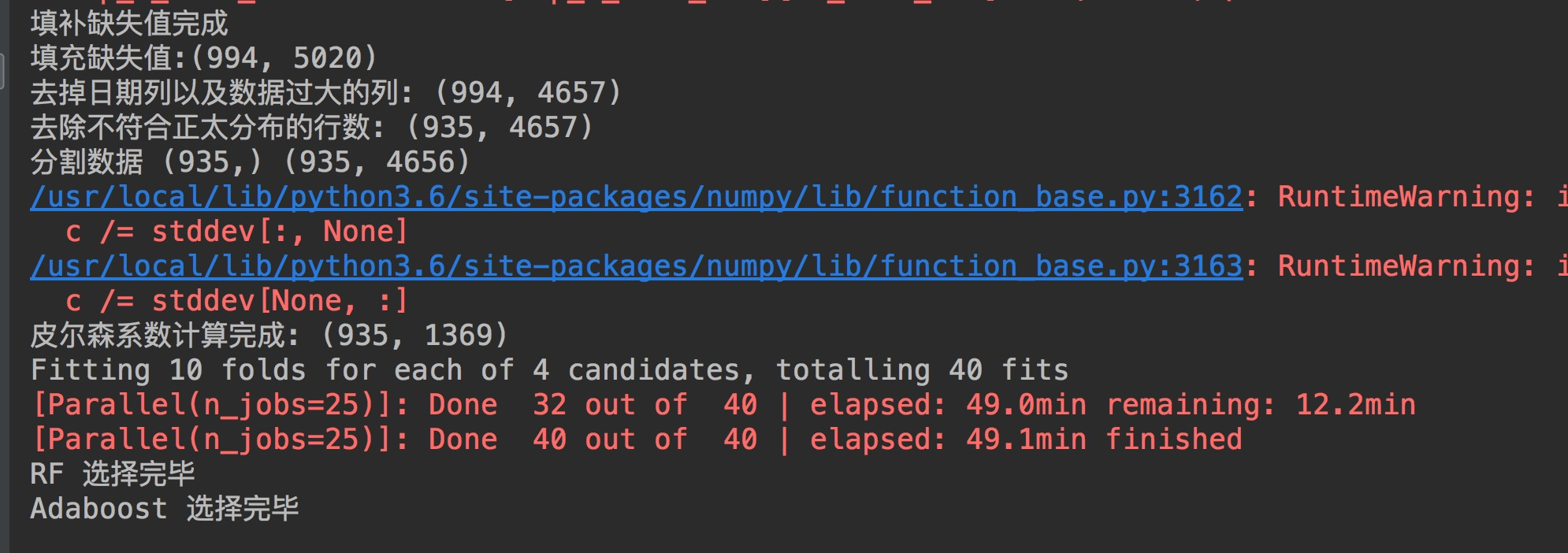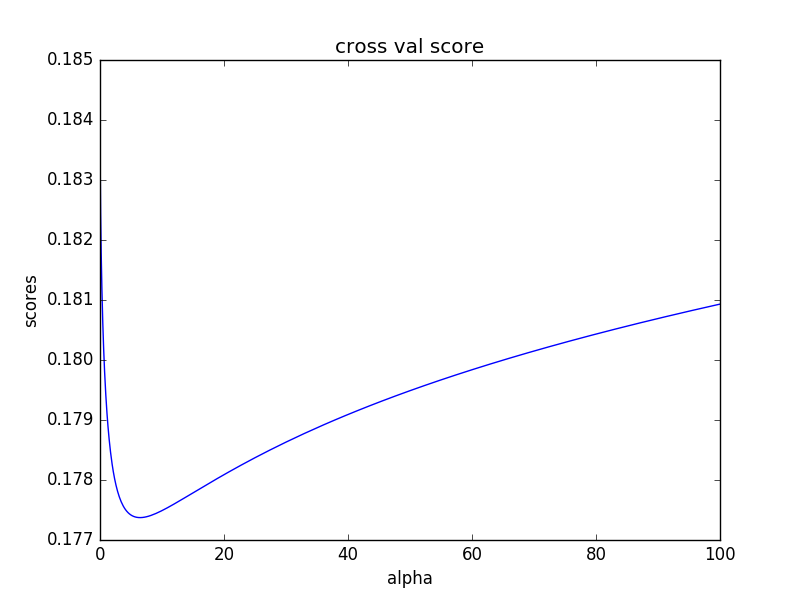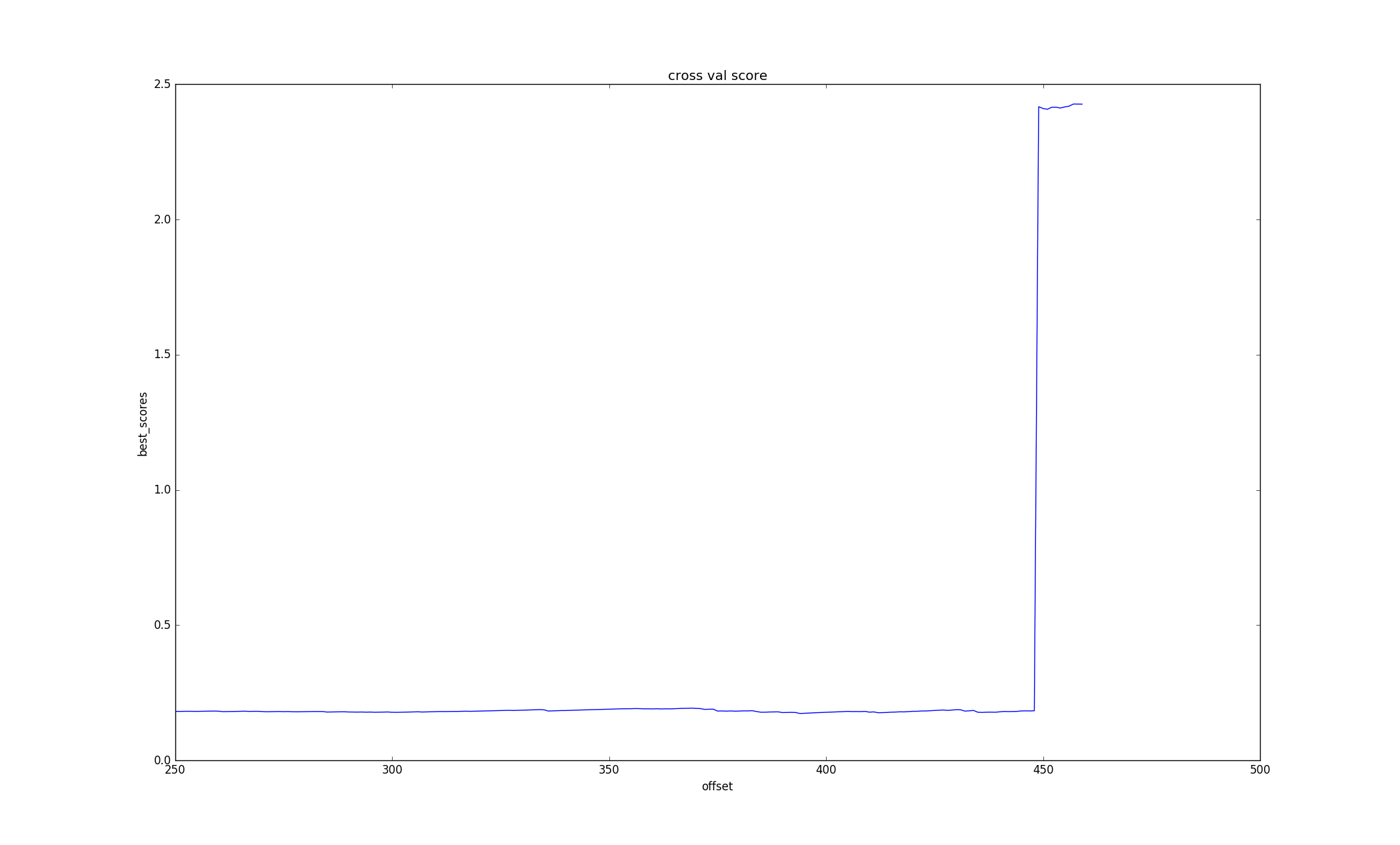1.比赛链接：天池工业AI大赛-智能制造质量预测，最终排名89/2529

2.Github链接：IntelligentMachine## 概述

• 该比赛分为初赛、复赛和决赛，很遗憾，我只走到了复赛。初赛、复赛都是A/B榜切换机制，平时是A榜单数据验证，一天只能验证一次，B榜在最后两天放出来，只有两次提交机会，对A榜单过拟合的会哭得很惨…初赛只有500条训练数据，却有8000多列，需要预测100条数据，复赛是700×5000这样的维度，主办方解释是因为这些数据非常难采集。。。所以我的第一感觉就是，这是一个很不靠谱的比赛，事实也证明，运气成分很大。
比赛中，我的主要目的是学习。在此之前，关于机器学习只手动实现过KNN,ANN, 朴素贝叶斯等简单的算法，其他的算法都只停留在了解一丢丢原理的层面上，从没有在程序中实践过，连sklearn都还没安装。通过近一个月的比赛后，算是熟练掌握了pandas\numpy\sklearn\xgboost等库的调用。。。
因为缺少经验，所以在比赛中我大量阅读别人的博客、代码，也向一些有经验的同学请教（感谢满绩王的亲情指导），这样做确实比我瞎尝试要好得多。
代码主要分为两部分，特征工程 + 机器学习，和大多数博客一样，特征工程占据了绝大多数时间，而对于这样一个 500 x 8000的数据，特征工程简直就是玄学。

## 比赛中所用的算法和技术回顾

### 特征工程

• 去除错误列

去除数据中缺失值过多的列或行，参数得自己调整。

• 去除错误行

对于不符合正态分布3 sigmoid的行（根据正太分布原理，数值分布在（μ—3σ,μ+3σ)中的概率为0.9974），超过一定数量就删除该行。这部分代码如下:

```# 去除不符合正太分布的行
def remove_wrong_row(data):
# 计算每一列数据的上界
upper = data.mean(axis=0) + 3 * data.std(axis=0)
# 计算每一列数据的下界
lower = data.mean(axis=0) - 3 * data.std(axis=0)
# 计算每一行中超过上界的数值的数量
wrong_data1 = (data > upper).sum(axis=1).reset_index()
wrong_data1.columns = ['row', 'na_count']
# 参数是经过调试的
# 记录数量超过一定值的行数
wrong_row1 = wrong_data1[wrong_data1.na_count >= 40].row.values
# 计算每一行中超过下界的数值的数量
wrong_data2 = (data < lower).sum(axis=1).reset_index()
wrong_data2.columns = ['row', 'na_count']
wrong_row2 = wrong_data2[wrong_data2.na_count >= 95].row.values
wrong_row = np.concatenate((wrong_row1, wrong_row2))
# 去除不符合正太分布的行
data.drop(wrong_row, axis=0, inplace=True)
return data```
• 填补缺失值

由于缺失的值比较多，单纯的使用fillna()的效果不是很好，在满绩王的启发下，写了KNN 近邻填充。就是利用欧式距离上距离每一行最近的K行在某一列的平均值来填充该行在该列的缺失值。具体实现代码如下（我已经尽量使用矩阵的算法来计算距离，避免使用for循环，但代码中还是使用了一层for循环，如果哪位大佬知道更好的方式，请告诉我）：

```def knn_fill_nan(data, K):

# 计算每一行的空值，如有空值则进行填充，没有空值的行用于做训练数据
data_row = data.isnull().sum(axis=1).reset_index()
data_row.columns = ['raw_row', 'nan_count']
# 空值行（需要填充的行）
data_row_nan = data_row[data_row.nan_count > 0].raw_row.values

# 非空行 原始数据
data_no_nan = data.drop(data_row_nan, axis=0)

# 空行 原始数据
data_nan = data.loc[data_row_nan]
for row in data_row_nan:
data_row_need_fill = data_nan.loc[row]
# 找出空列，并利用非空列做KNN
data_col_index = data_row_need_fill.isnull().reset_index()
data_col_index.columns = ['col', 'is_null']
is_null_col = data_col_index[data_col_index.is_null == 1].col.values
data_col_no_nan_index = data_col_index[data_col_index.is_null == 0].col.values
# 保存需要填充的行的非空列
data_row_fill = data_row_need_fill[data_col_no_nan_index]

# 广播，矩阵 - 向量
data_diff = data_no_nan[data_col_no_nan_index] - data_row_need_fill[data_col_no_nan_index]
# 求欧式距离
# data_diff = data_diff.apply(lambda x: x**2)
data_diff = (data_diff ** 2).sum(axis=1)
data_diff = data_diff.apply(lambda x: np.sqrt(x))
data_diff = data_diff.reset_index()
data_diff.columns = ['raw_row', 'diff_val']
data_diff_sum = data_diff.sort_values(by='diff_val', ascending=True)
data_diff_sum_sorted = data_diff_sum.reset_index()
# 取出K个距离最近的row
top_k_diff_row = data_diff_sum_sorted.loc[0:K - 1].raw_row.values
# 根据row 和 col值确定需要填充的数据的具体位置（可能是多个）
# 填充的数据为最近的K个值的平均值
top_k_diff_val = data.loc[top_k_diff_row][is_null_col].sum(axis=0) / K

# 将计算出来的列添加至非空列
data_row_fill = pd.concat([data_row_fill, pd.DataFrame(top_k_diff_val)]).T
# print(data_no_nan.shape)
data_no_nan = data_no_nan.append(data_row_fill, ignore_index=True)
# print(data_no_nan.shape)
print('填补缺失值完成')
return data_no_nan```
• 去除日期列

在本比赛环境中，日期对生产误差应该没有影响，但是会影响我们的线性模型，所以删除

• 将非浮点数列转化为浮点数列或直接删除

主要是将数据值为字母、单词等列转换为浮点数，这些列主要是生产工具的名称，可能会有影响，在经过尝试之后，发现直接删除这些列效果更好一丢丢。

• 去除皮尔森系数在[-0.1, 0.1]之间的特征列

上过概率统计的应该还记得它，它描述了两个数据之间的线形相关性，值属于[-1, 1]，-1表示完全负相关，1表示完全相关，0表示完全不相关。这里去除了绝对值小于0.1的特征，也就是几乎没什么关系的列。

• 特征选择

```def ensemble_model_feature(X, Y, top_n_features):
features = list(X)
# 随机森林
rf = ensemble.RandomForestRegressor()
rf_param_grid = {'n_estimators': , 'random_state': [2, 4, 6, 8]}
rf_grid = GridSearchCV(rf, rf_param_grid, cv=10, verbose=1, n_jobs=25)
rf_grid.fit(X, Y)
top_n_features_rf = get_top_k_feature(features=features, model=rf_grid, top_n_features=top_n_features)
print('RF 选择完毕')
abr_grid = GridSearchCV(abr, rf_param_grid, cv=10, n_jobs=25)
abr_grid.fit(X, Y)
top_n_features_bgr = get_top_k_feature(features=features, model=abr_grid, top_n_features=top_n_features)
# ExtraTree
etr = ensemble.ExtraTreesRegressor()
etr_grid = GridSearchCV(etr, rf_param_grid, cv=10, n_jobs=25)
etr_grid.fit(X, Y)
top_n_features_etr = get_top_k_feature(features=features, model=etr_grid, top_n_features=top_n_features)
print('ETR 选择完毕')
# 融合以上三个模型
features_top_n = pd.concat([top_n_features_rf, top_n_features_bgr, top_n_features_etr],
ignore_index=True).drop_duplicates()
print(features_top_n)
print(len(features_top_n))
return features_top_n```
• 数据规范化（Normalization）

这是一项基本操作，由于数据与数据之间值的差距特别大，为了减少误差，须通过规范化，将所有数据的值都映射到同一范围内。规范化的具体实现有很多种，我最终使用的是sklearn.preprocess.scale()。另外，如果自己实现的话，代码如下：

```# 自定义规范化数据
def normalize_data(data):
# 最大最小值规范化
return data.apply(lambda x: (x - np.min(x)) / (np.max(x) - np.min(x)))
# z-socre 规范化
# return data.apply(lambda x: (x - np.average(x)) / np.std(x))```
• 至此，特征工程的所有工作就完成了。最终特征的维数在170左右，根据参数的不同会有一些变化。### 机器学习

• 最小二乘线性拟合， sklearn.linear_model.LinearRegression

• 最小二乘线性拟合 + L2正则，sklearn.linear_model.Ridge

• Ridge + Bagging 集成学习

• xgboost及调参

xgboost在很多文章中都被推崇，初赛中也使用了xgboost，但是还不怎么会调参，所以效果不是很好。在复赛A榜阶段，使用xgboost取得了不错的成果，并通过将xgboost与Bagging进一步融合，再将以前提提交的线上验证比较好的答案融合，得到了A榜最好的一次成绩（其实也很糟糕…)

### 总结

• 1.这次比赛中所用的算法都是比较常规的，由于数据集的特点（纬度太高，样本太少），几乎没有关注数据本身的意义
• 2.这个比赛很玄学，但真的很佩服那些A/B榜切换时几乎没怎么变化的大佬
• 3.多使用matplotlib.pyplot对各种样本进行绘图，直观的观察数据往往能发现一些神奇的东西• 3.还是继续好好学习一下再玩比赛吧

### 1 条评论#### william · 2019年12月7日 下午3:46

LGBMRegressor(boosting_type=’gbdt’, class_weight=None,
colsample_bytree=0.8686257629764181,
learning_rate=0.0247189003115119, max_depth=3, min_child_samples=20,
min_child_weight=24.791355759408724,
min_split_gain=0.012043335959975065, n_estimators=100, n_jobs=-1,
num_leaves=15, objective=’regression’, random_state=None,
reg_alpha=0.04837875509789402, reg_lambda=0.06890060081771218,
silent=True, subsample=0.8319777194152455, subsample_for_bin=200000,
subsample_freq=1)
The mse of prediction is: 0.040130501865083976